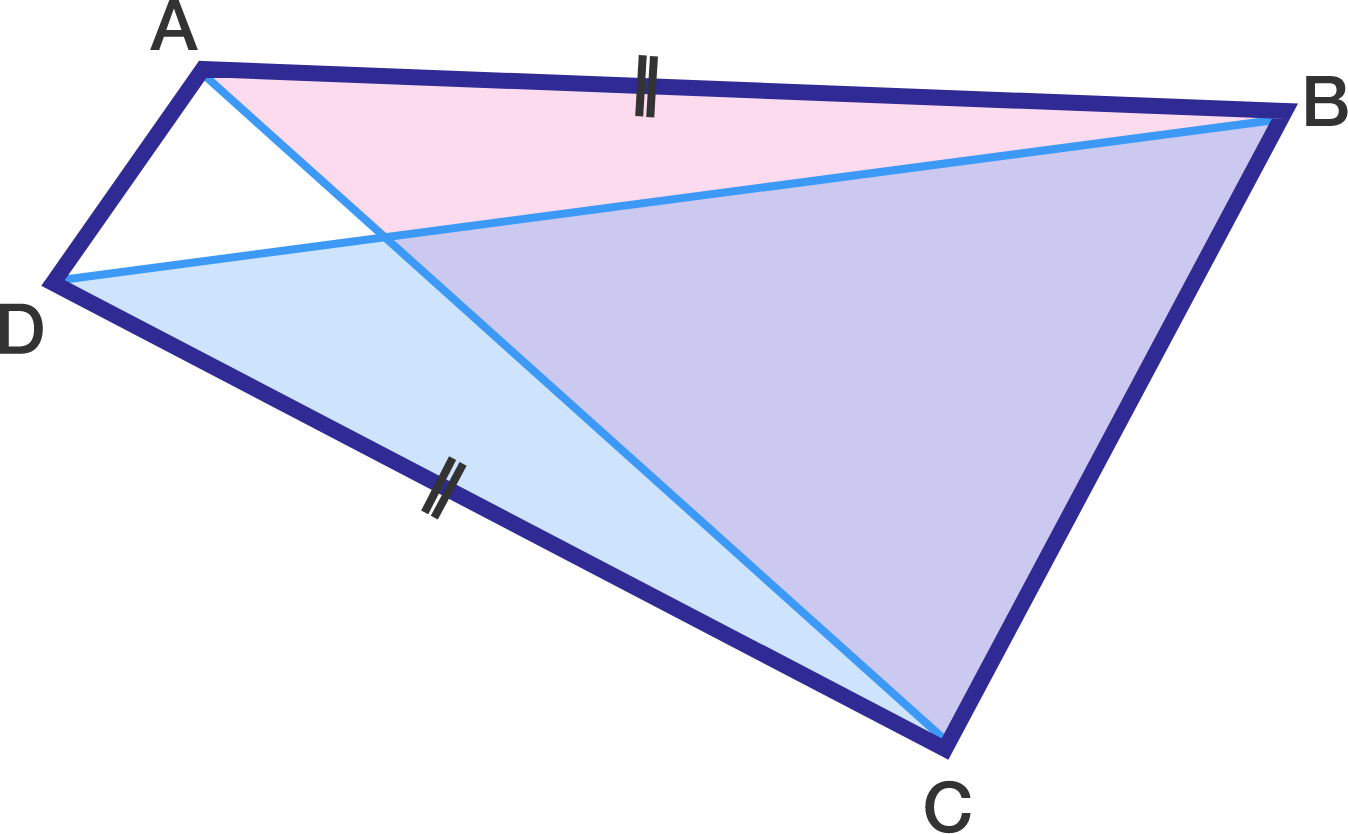Geometry Level 2Quadrilateral $ABCD$ has $AB=CD,$ and the acute angles $B$ and $C$ satisfy $\sin B = \frac{4}{9}$ and $\sin C= \frac{5}{6}.$ Find $\frac{\text{area of }\color{#D61F06}{\triangle ABC}}{\text{area of }\color{#3D99F6}{\triangle BDC}}.$